# Inter maths solutions for IIA Complex Numbers

Intermediate 2nd year maths chapter 1 solutions for some problems. These solutions are very easy to understand.

You can see the solutions for inter 1a

3. Matrices

You can also see the solutions for 1b

1. Locus

The straight line sa

Straight lines la

12. Rate measure

You can see the solutions for maths IIA inter

Complex numbers solutions for textbook

De Moivre’s Theorem textbook solutions

Exercise 3(b)

Exercise 3(c)

You can see solutions for Inter maths IIB

1. Circle

3. Parabola

4. Ellipse

# Intermediate 2nd year maths 2a chapter 1

## Complex numbers solutions inter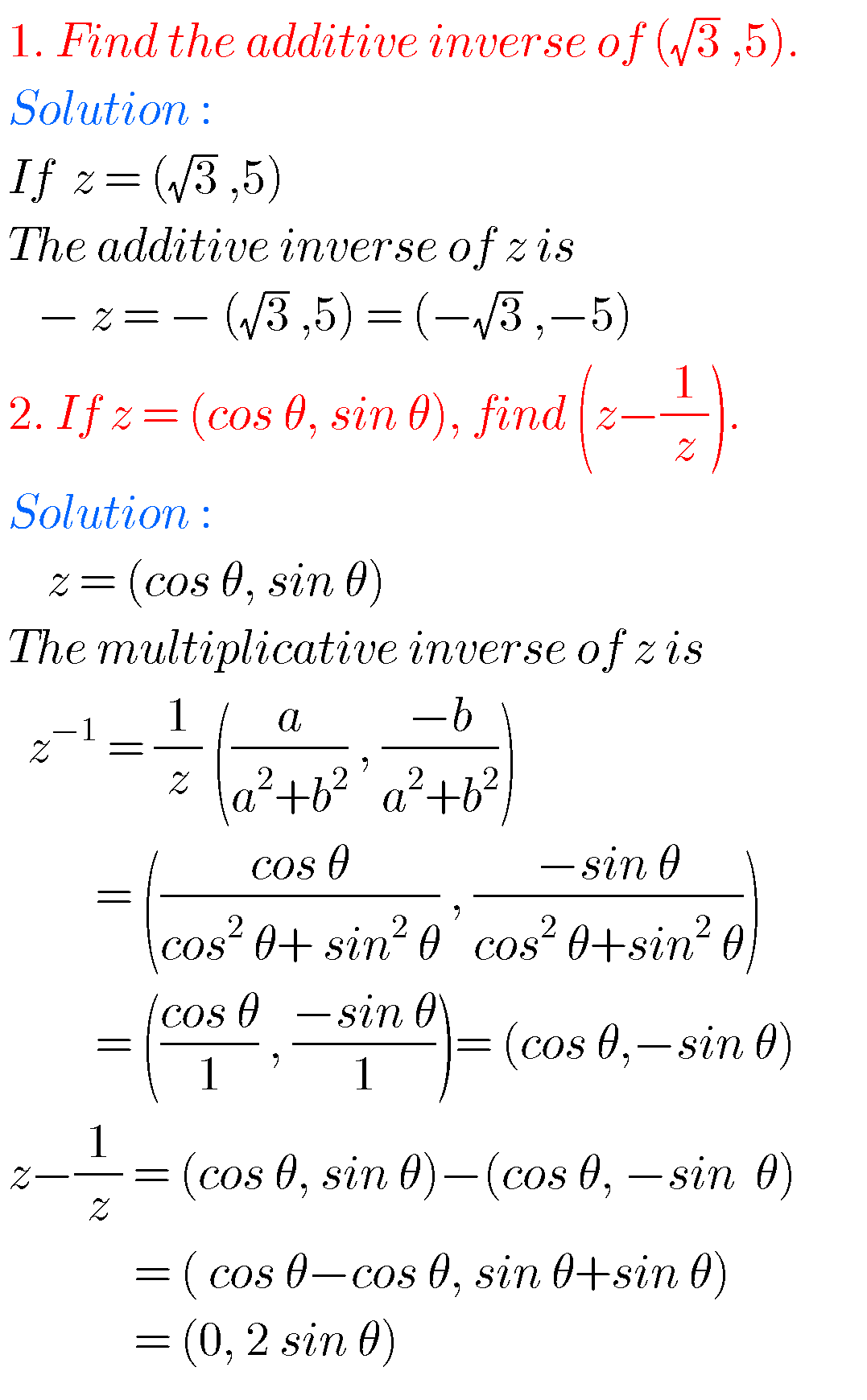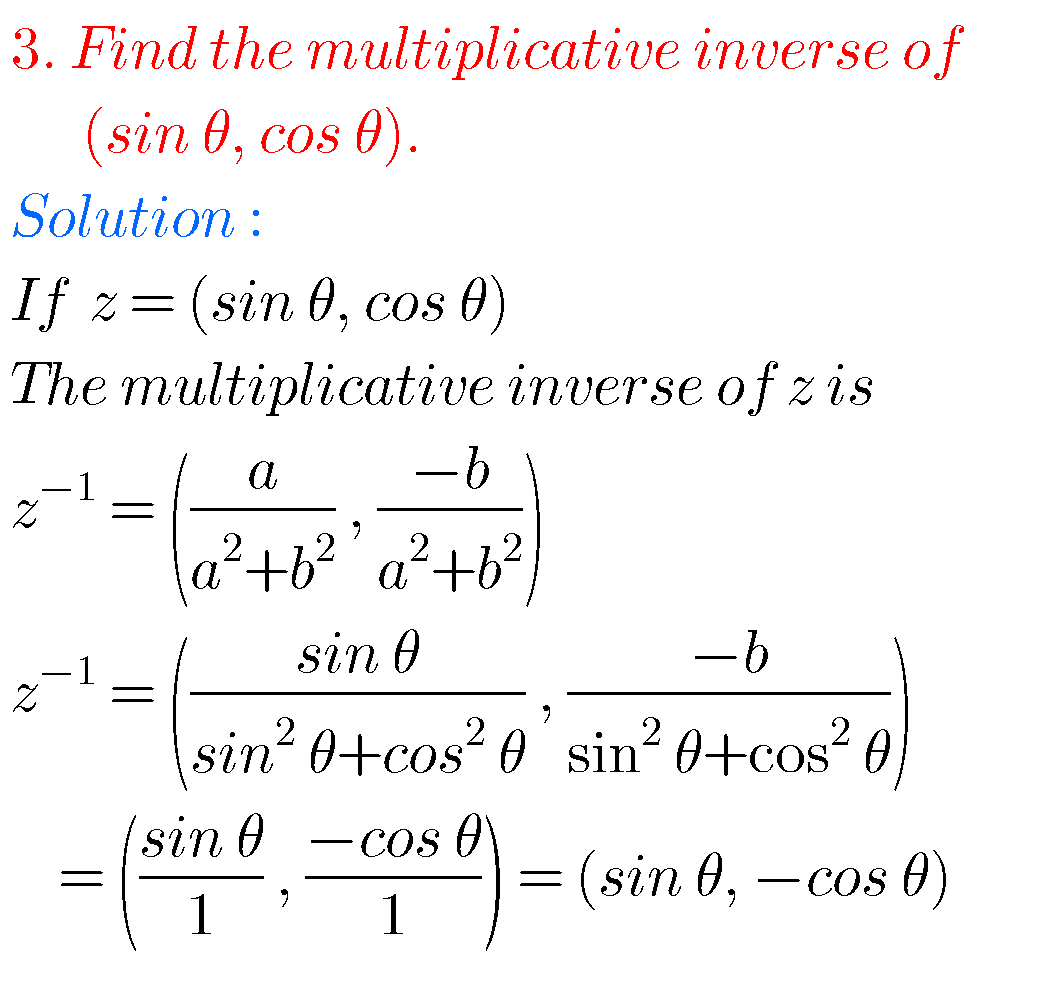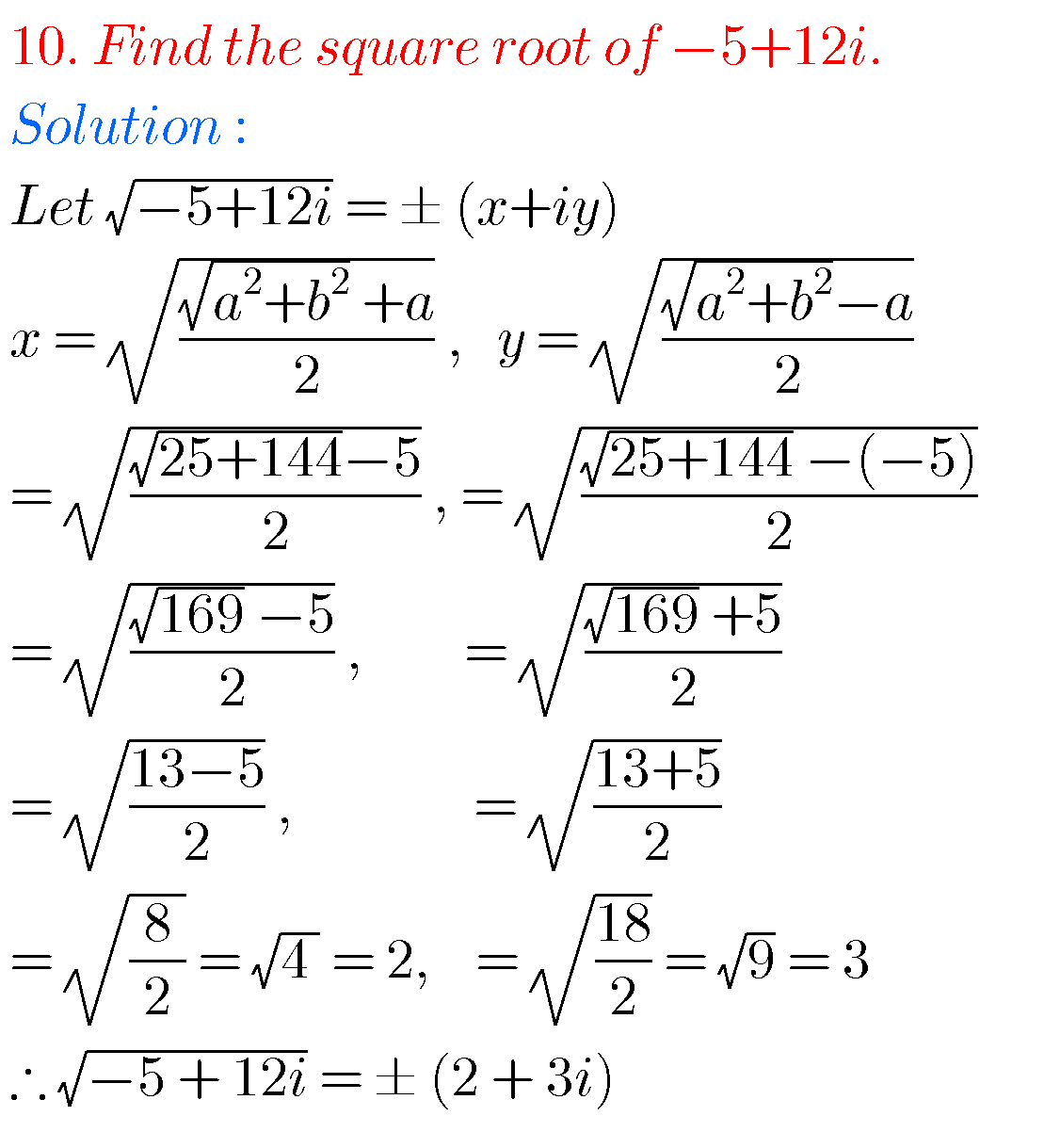##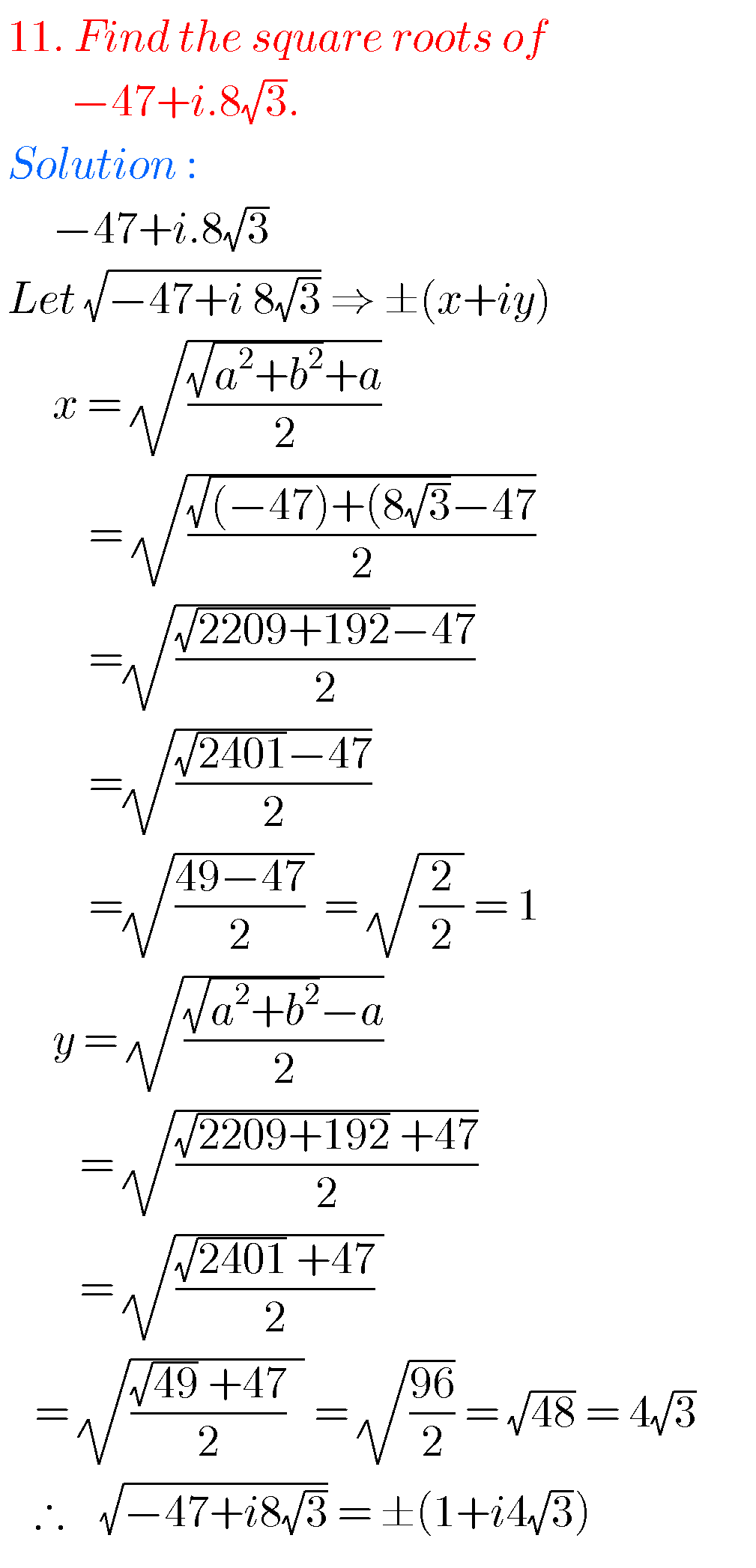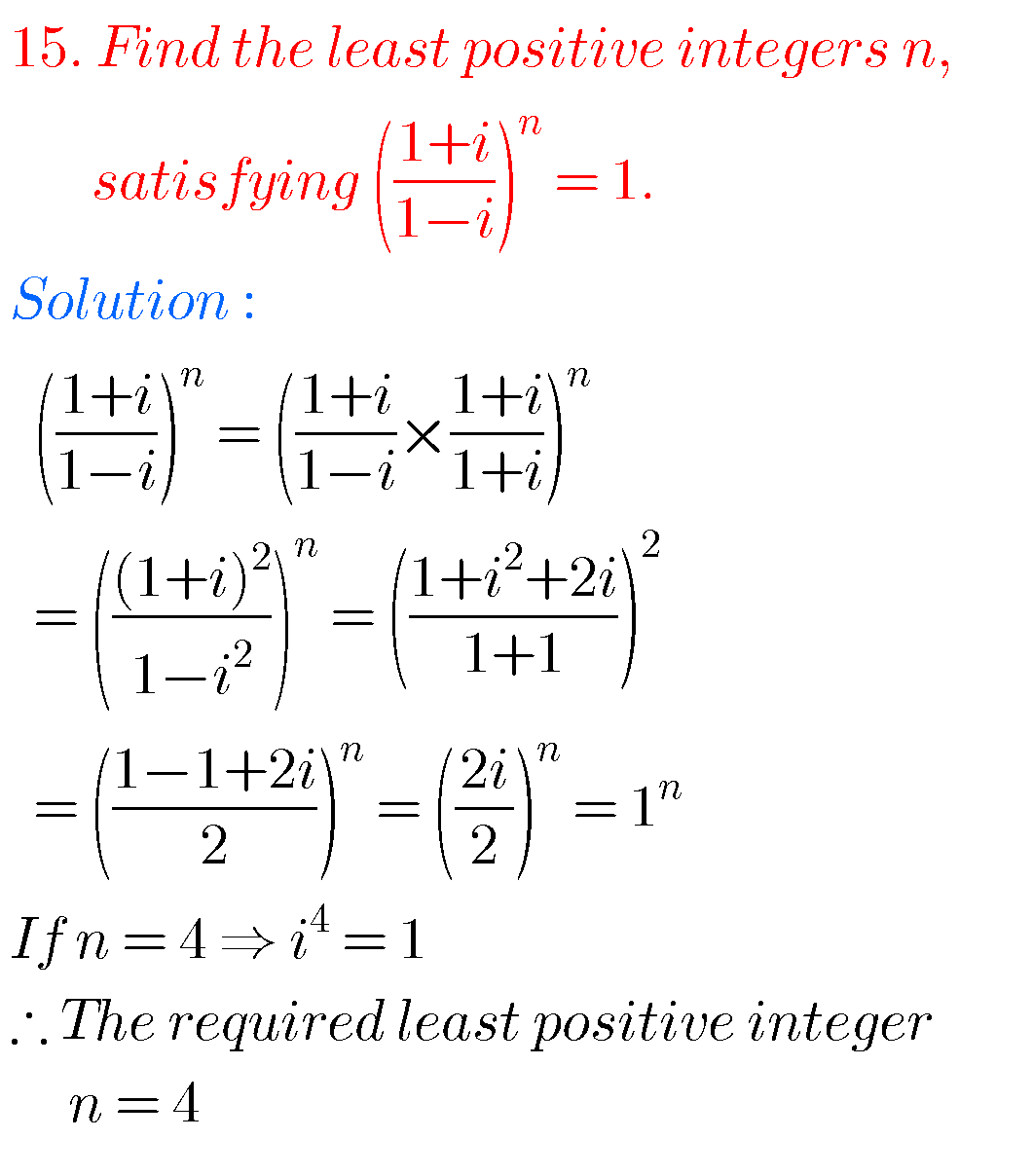##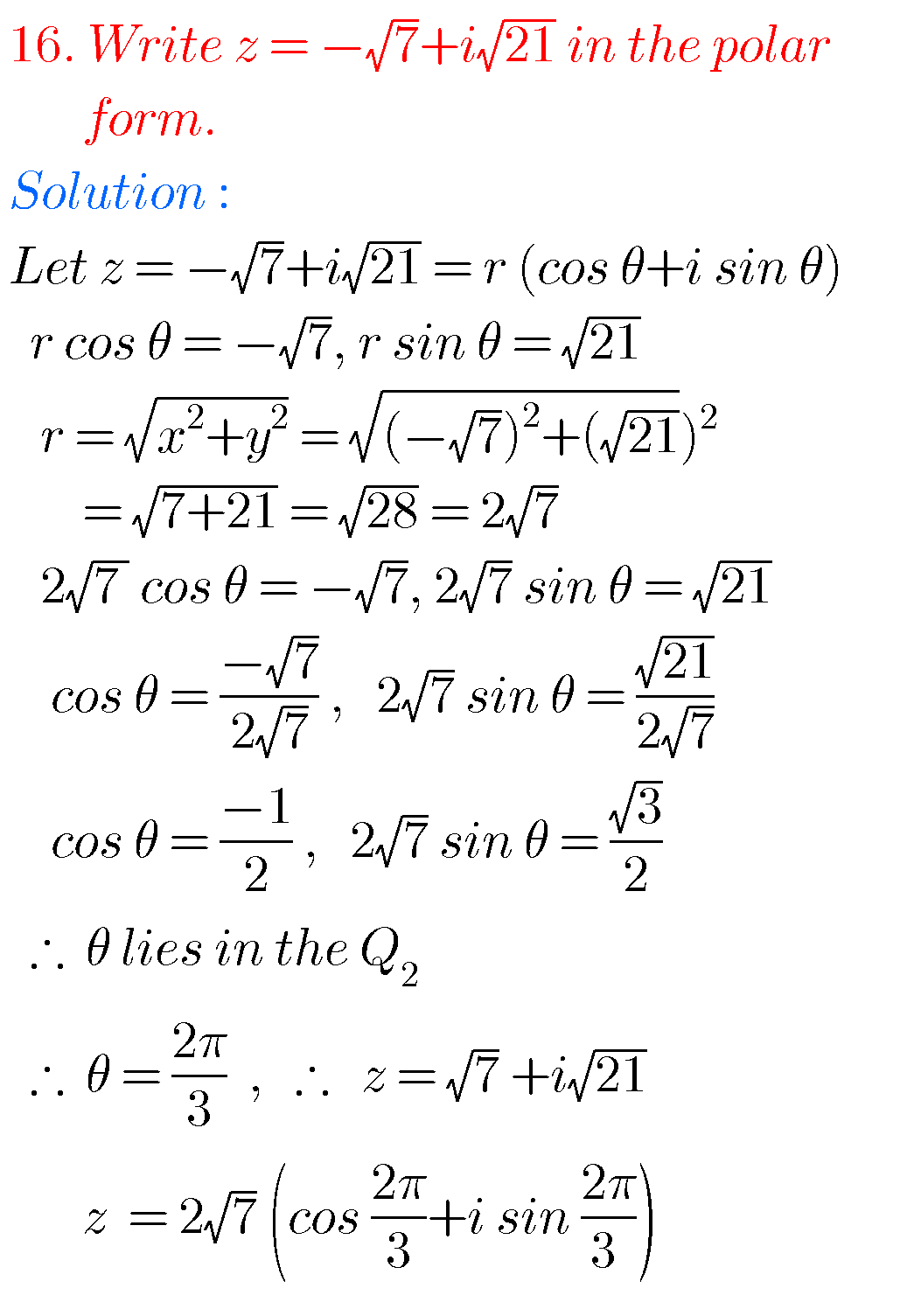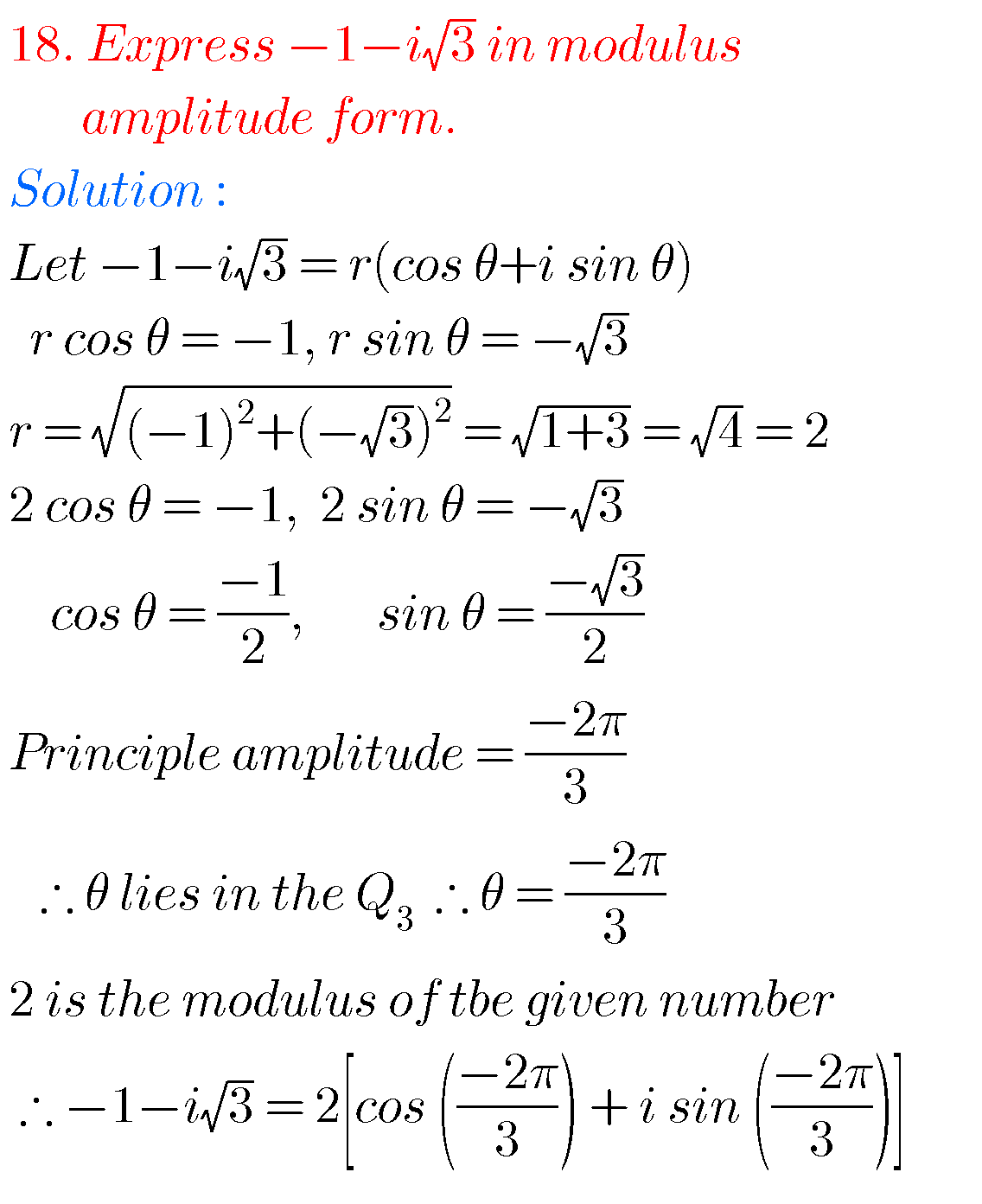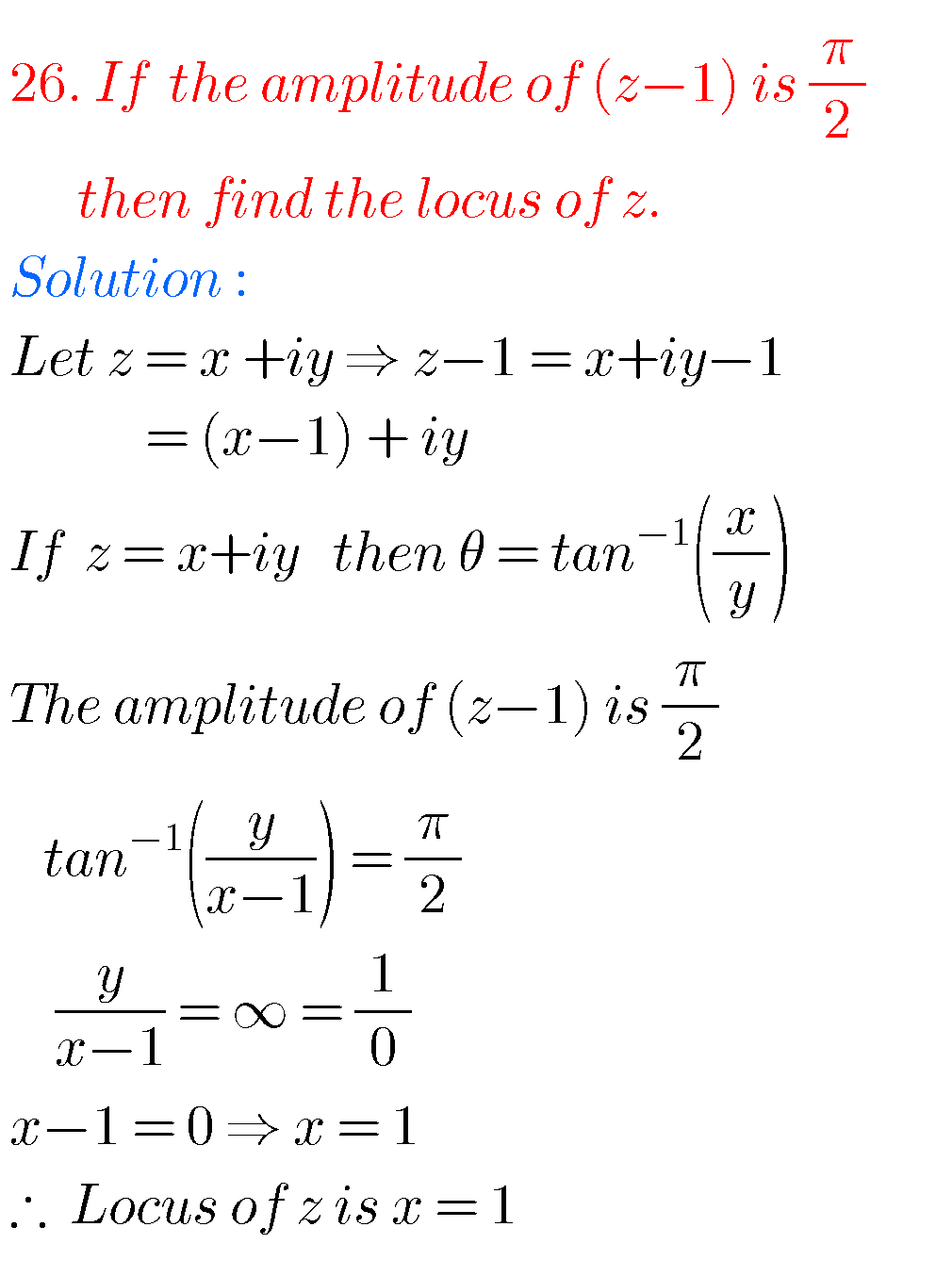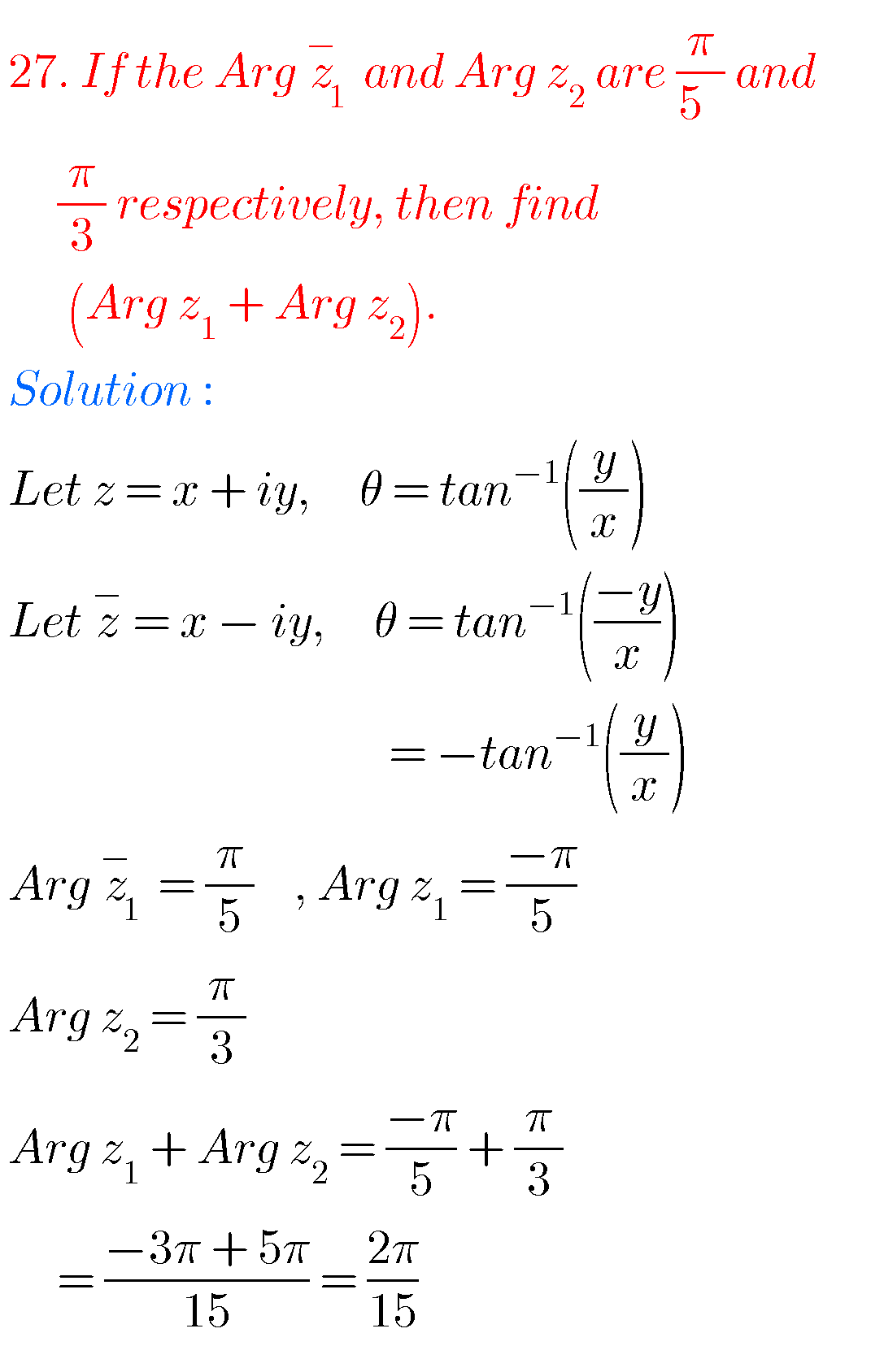##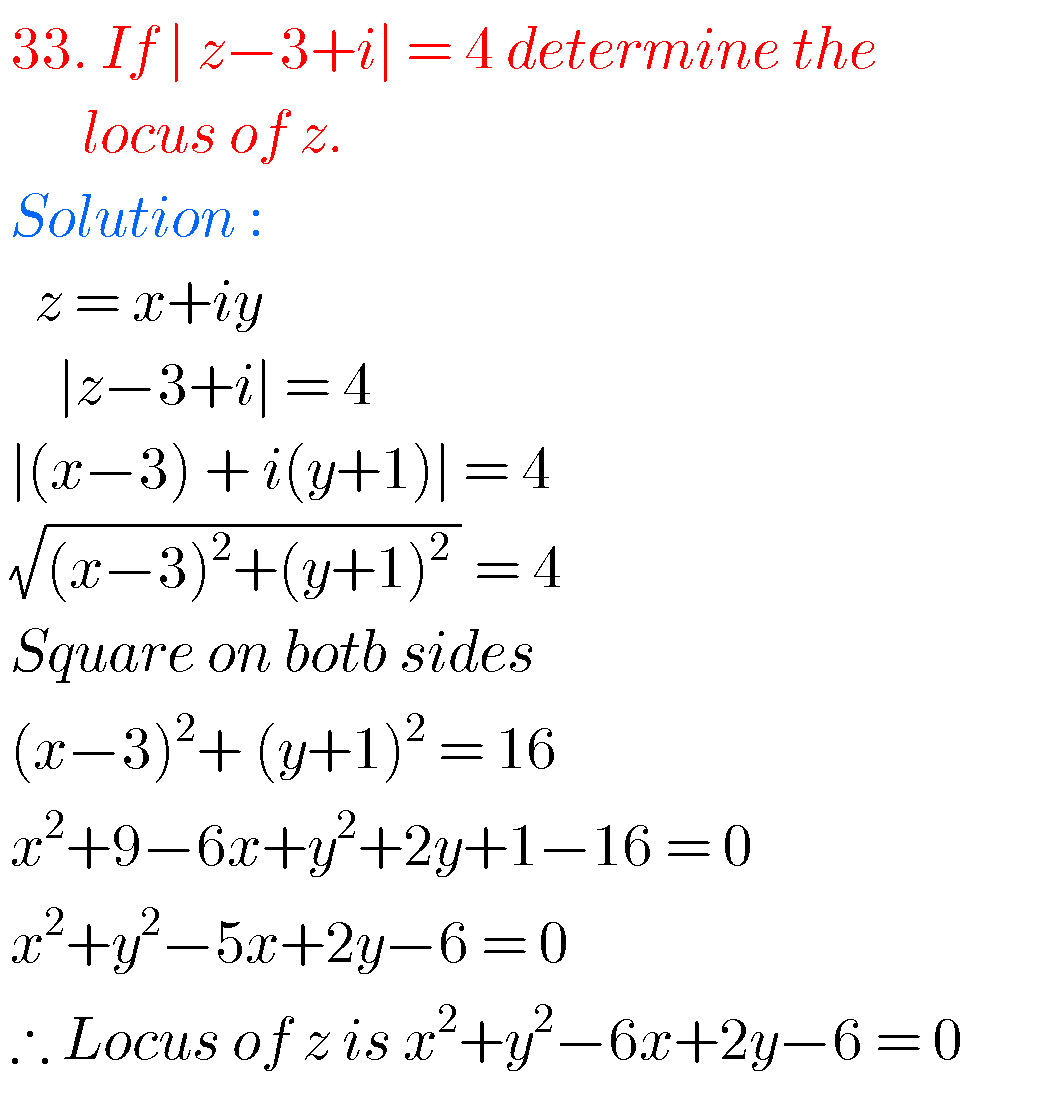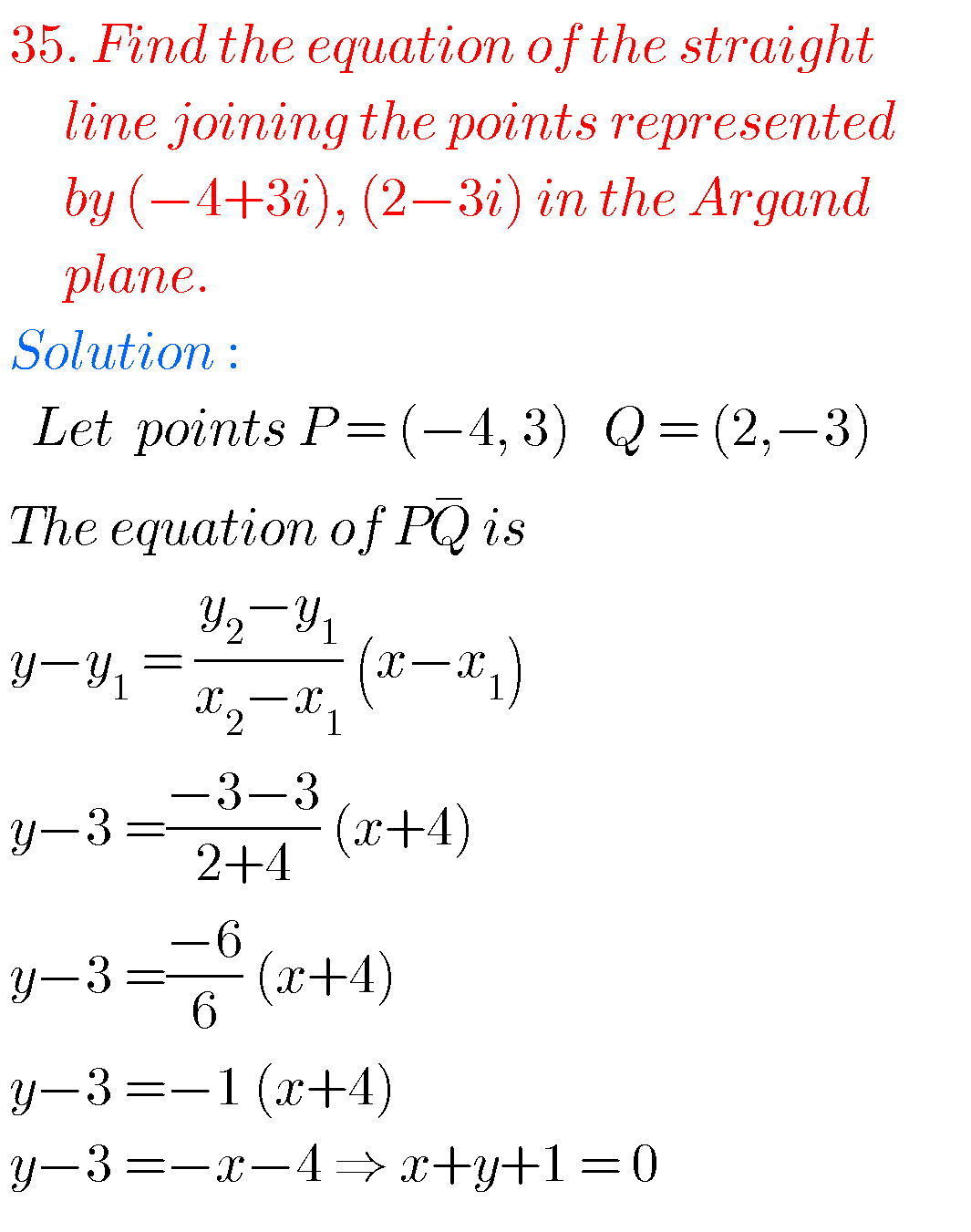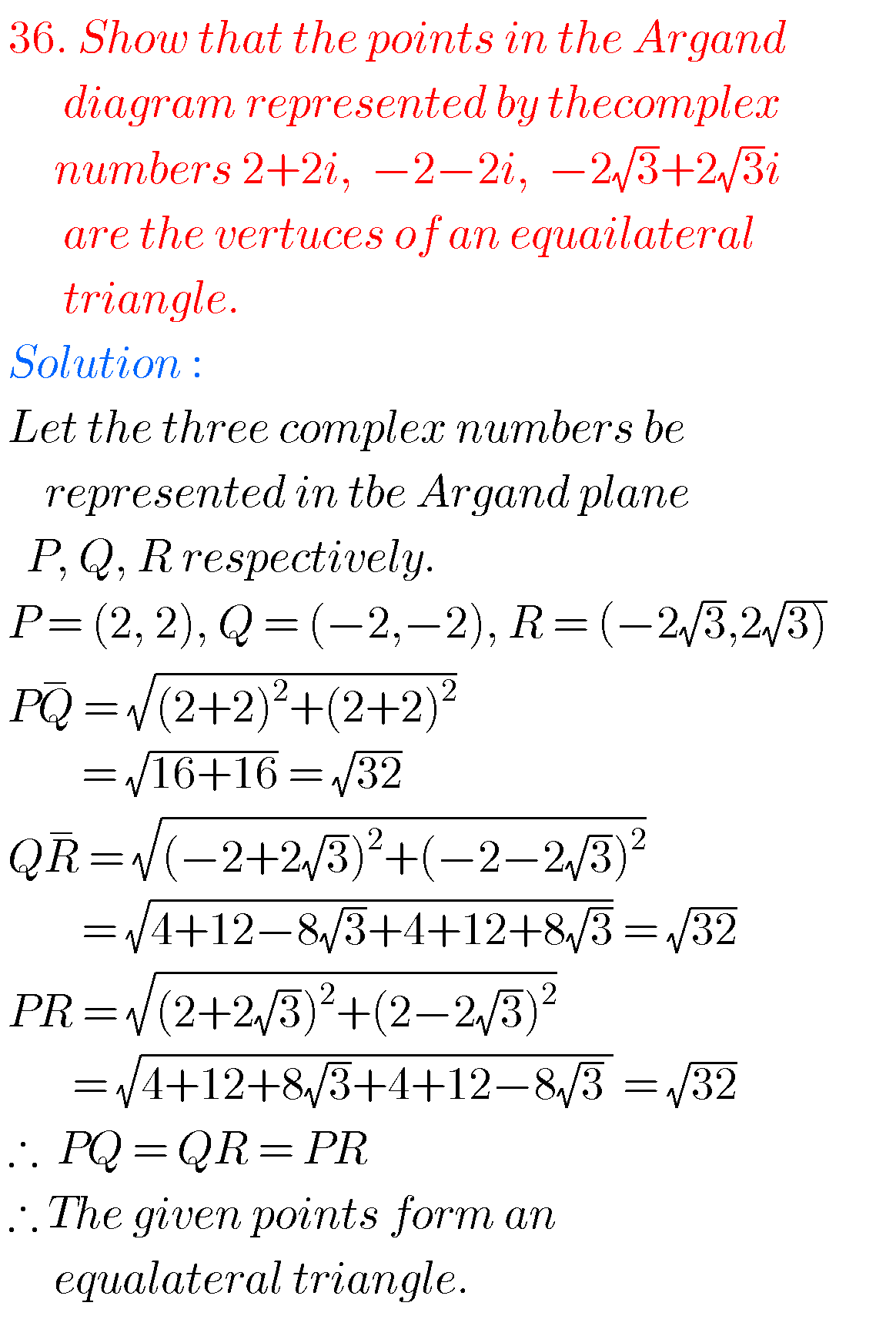#### Inter 2nd year maths 2a chapter 1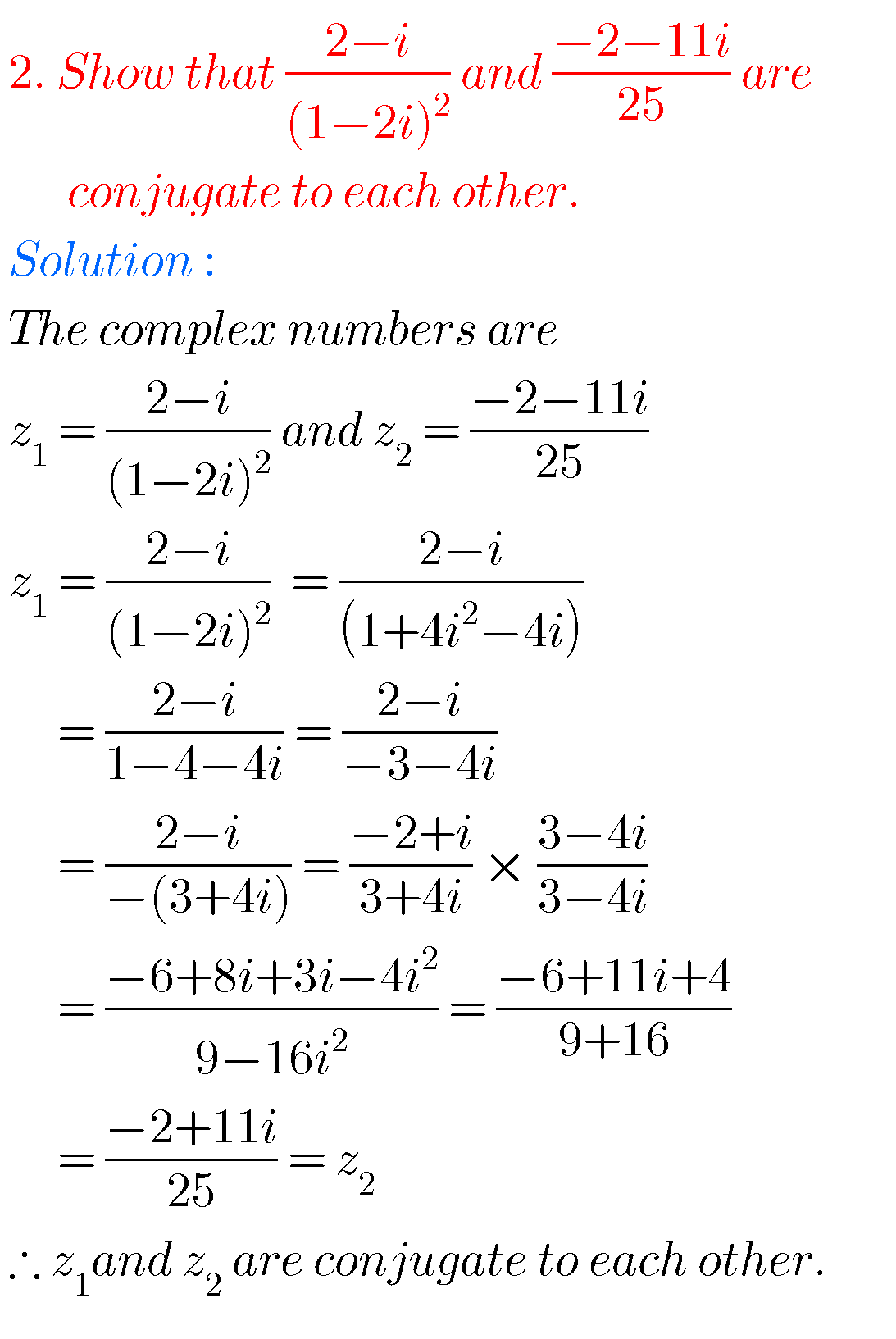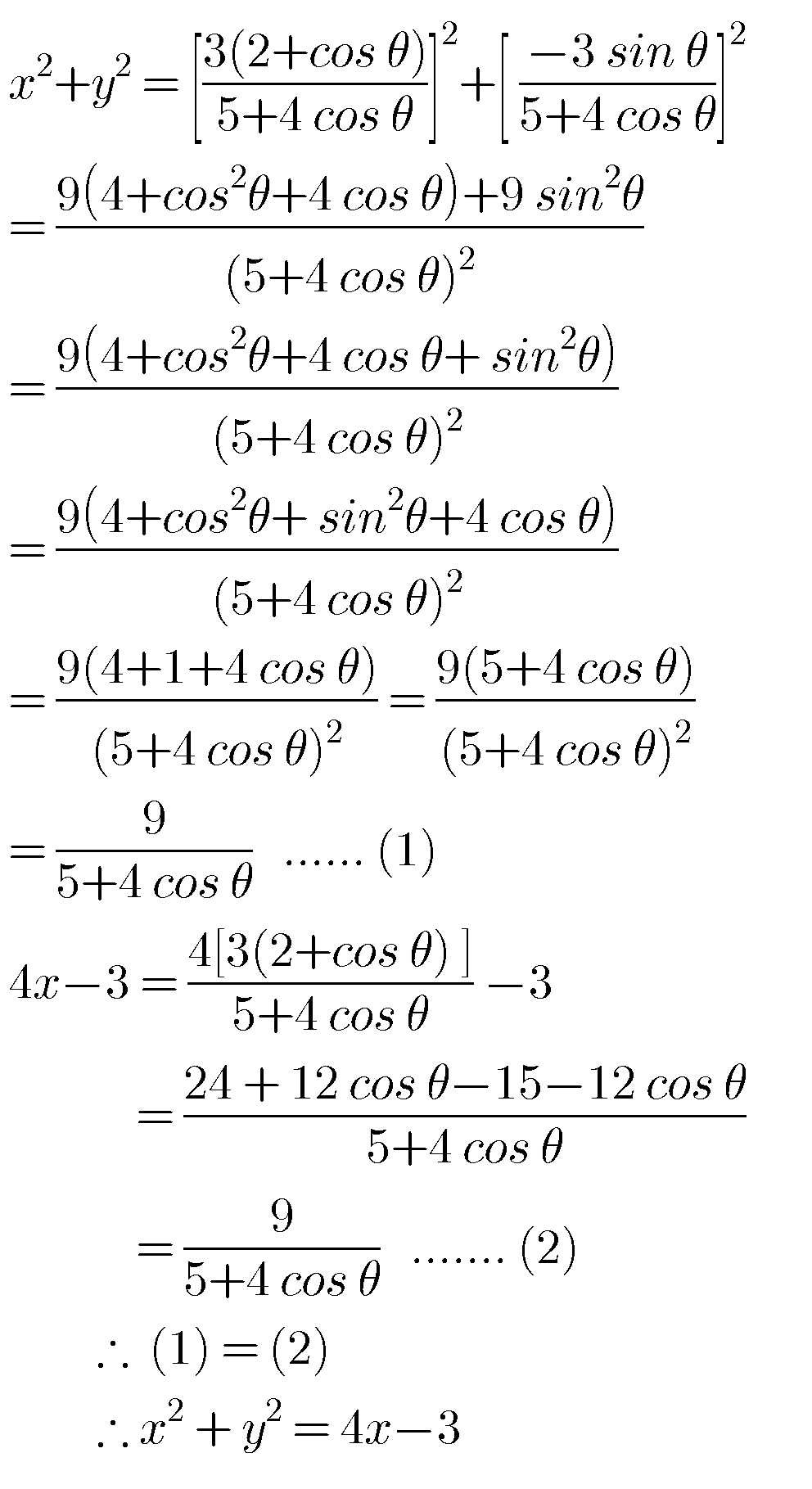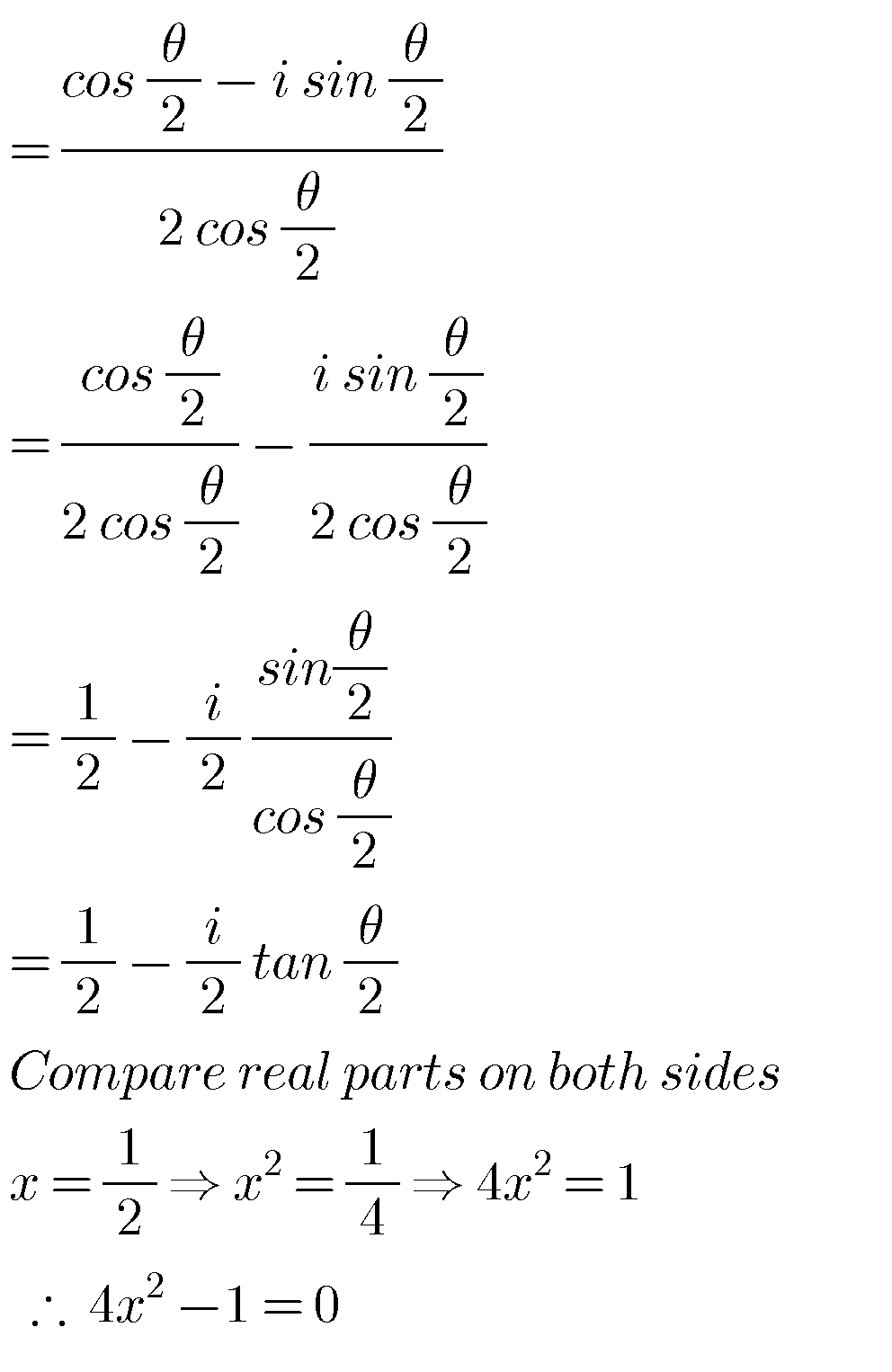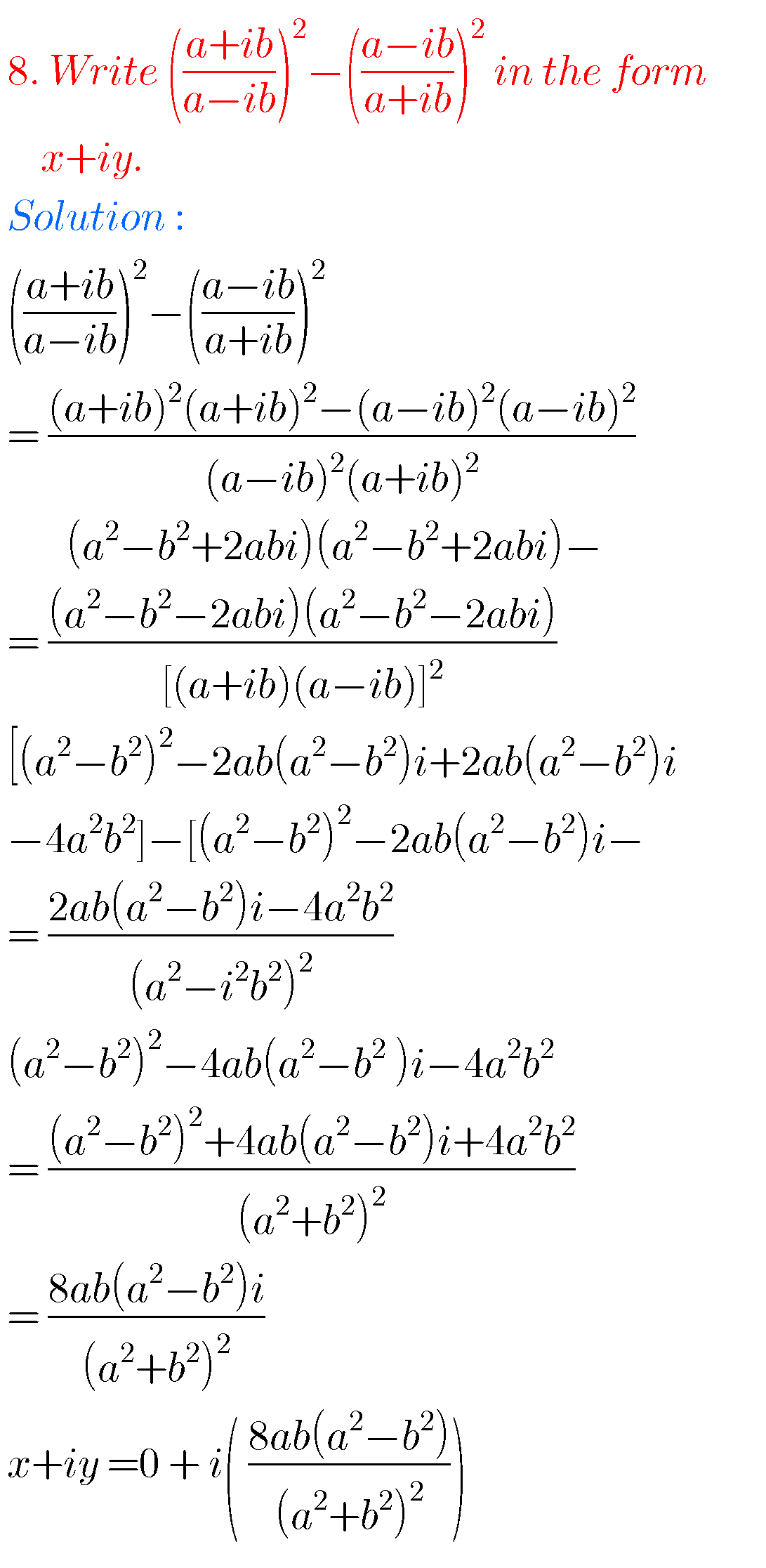### numbers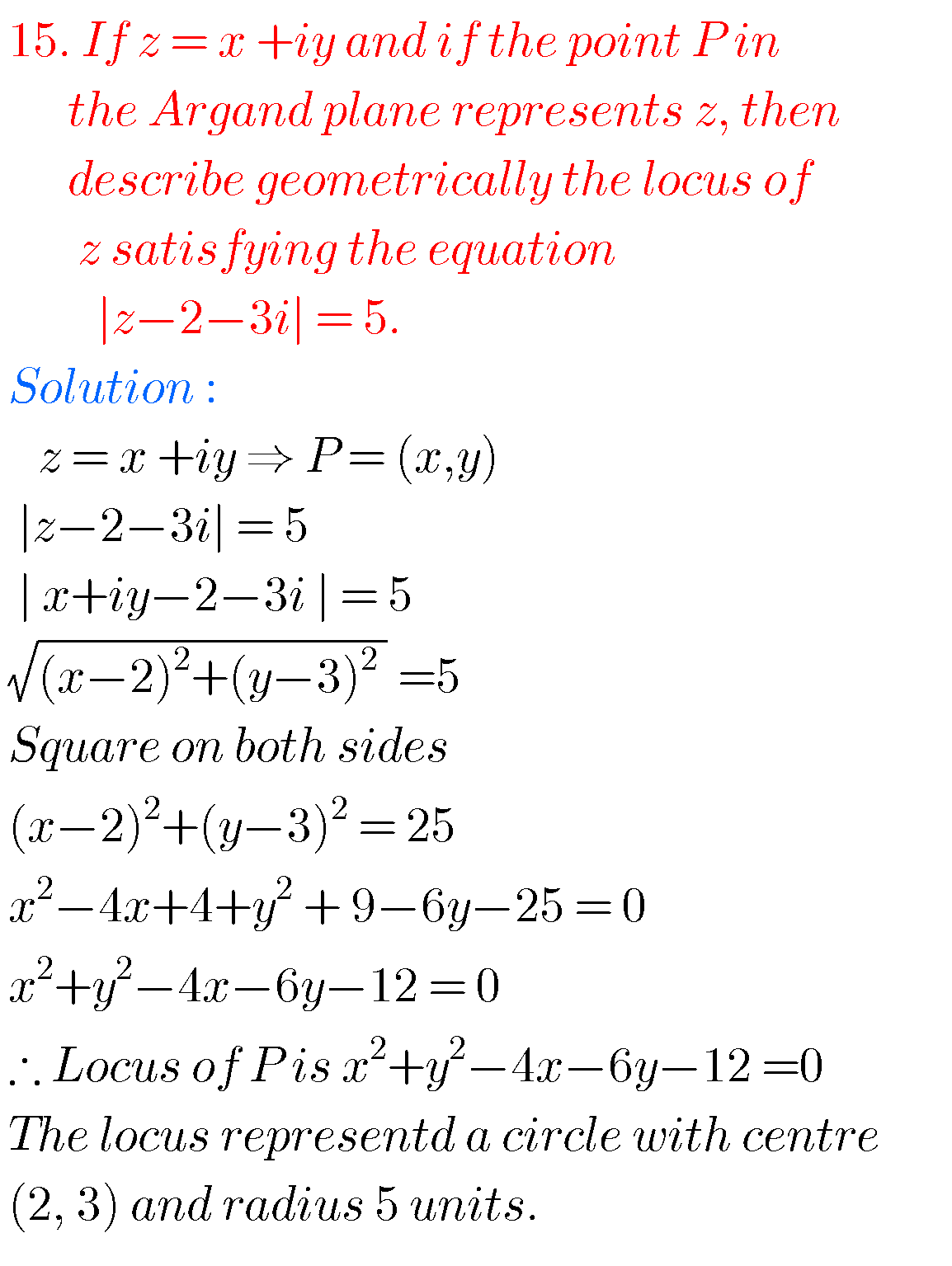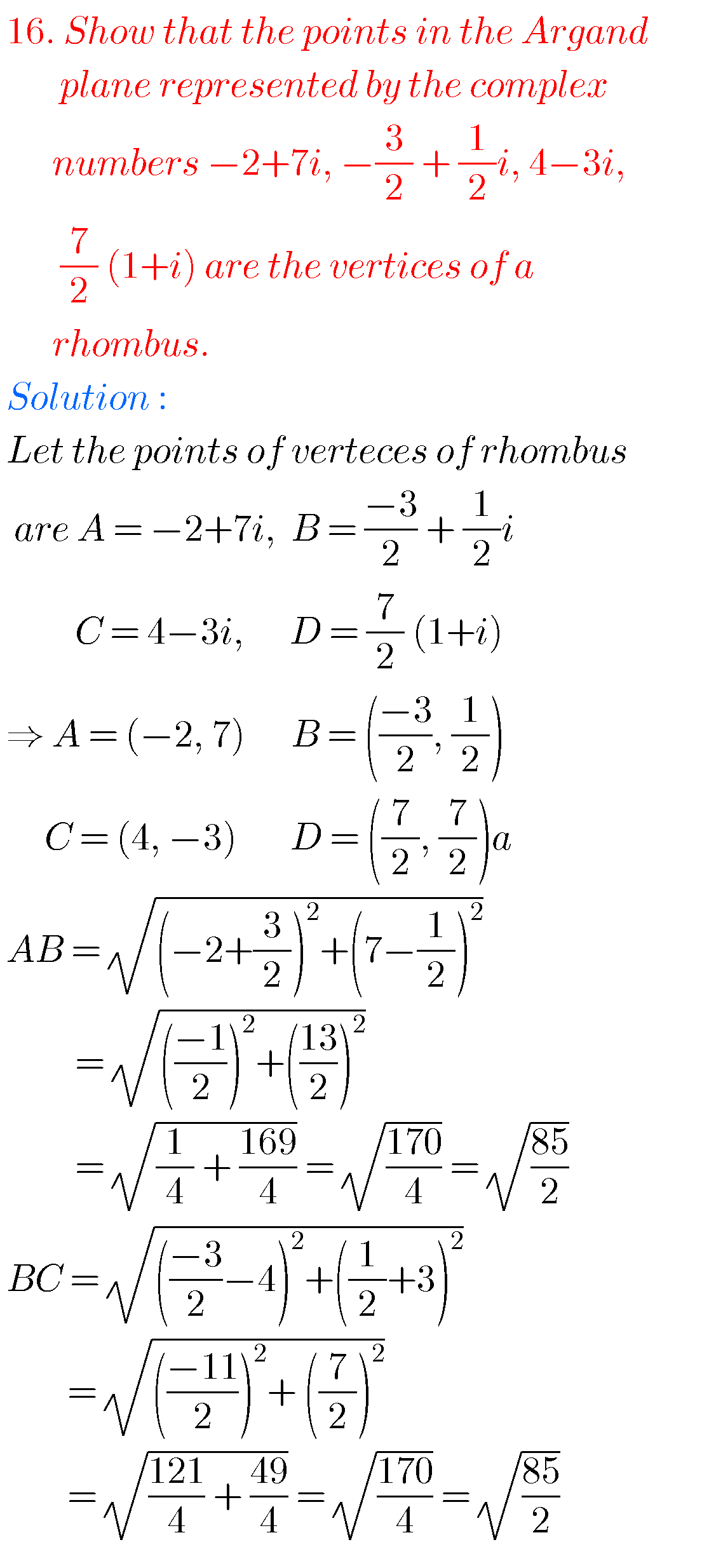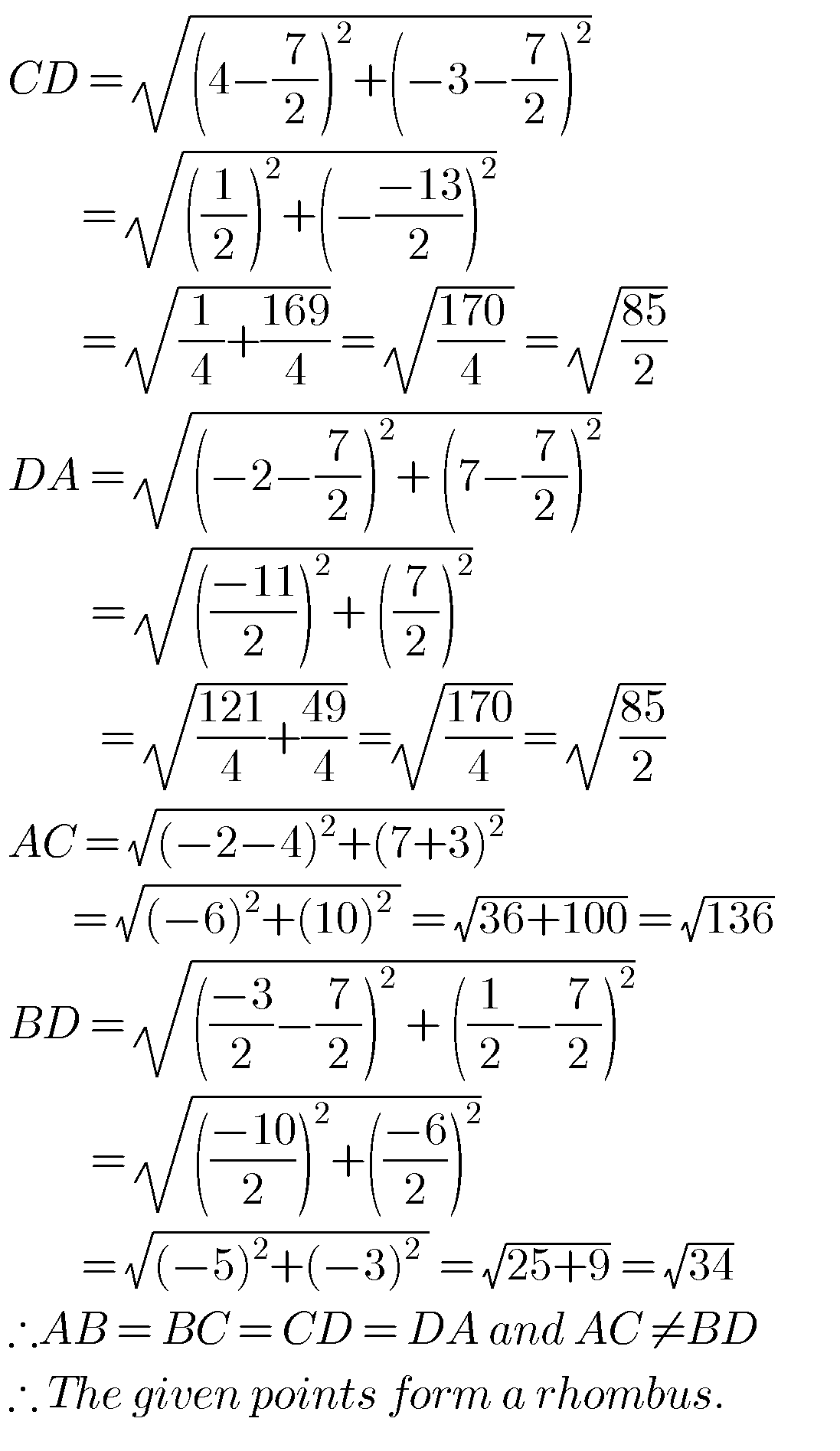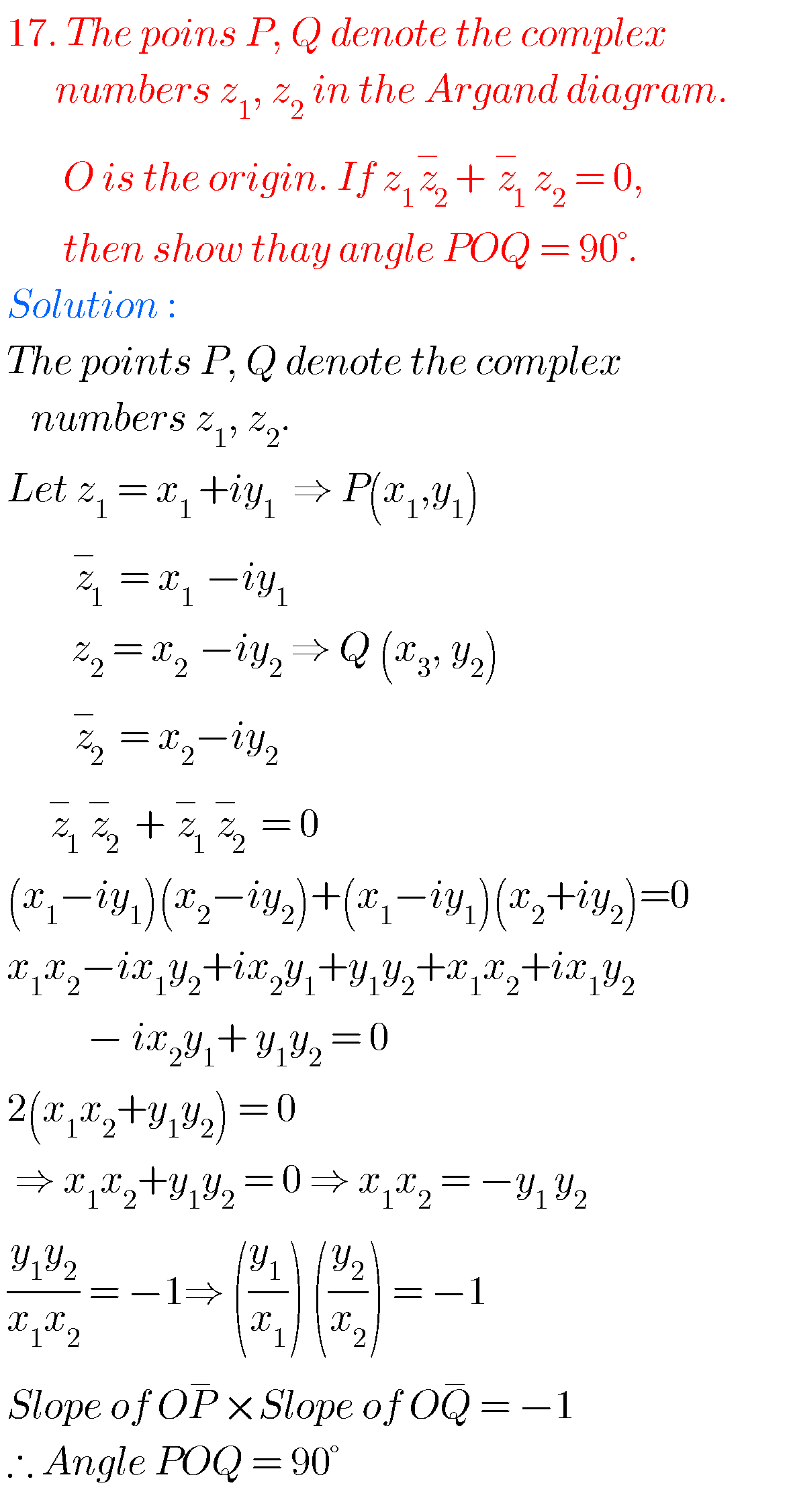### Intermediate IIA maths complex numbers solutions

Complex numbers solutions for textbook

Note: Observe the solutions and try them in your own methods.

Class 10 real numbers solutions

Ncert solutions for class 6 playing with numbers

1.Mohd ashraf nawaz November 4, 2020 at 12:19 pm - Reply

2.3.